# Multidimensional Arrays

The array described in this chapter is referred to as a one-dimensional array because the array consists of one series of elements. However, an array can have more than one series of elements. This is called a multidimensional array .

A multidimensional array consists of two or more arrays defined by sets of array elements, as shown in Figure 3-2. Each set of array elements is an array. The first set of array elements is considered the primary array, and the second and subsequent sets of array elements are considered subarrays.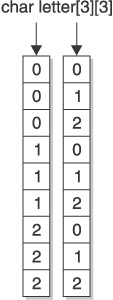Figure 3-2: A two-dimensional array is a multidimensional array consisting of two arrays.

There are two arrays in the multidimensional array shown in Figure 3-2. Each element of the first array points to a corresponding array. For example, letters  in Figure 3-2 points to the array beginning with array element letters where the zero is the first element of the second array.

Although you can create an array with any size multidimension, many programmers limit an array to two dimensions. Any size greater than two dimensions becomes unwieldy to manage.

An analogy we find helpful is visualizing a table (rows and columns ) for a two-dimensional array and a cube (or similar figure) for a three-dimensional array.

### Why Use a Multidimensional Array?

A multidimensional array can be useful to organize subgroups of data within an array. Let s say that a student has three grades, a mid- term grade, a final exam grade, and a final grade. You can store all three grades for an endless number of students in a two-dimensional array, as shown in Figure 3-3.Figure 3-3: All three grades can be stored in a multidimensional array.

Figure 3-3 declares a multidimensional array of integers. The first set of array elements contains three array elements, one for each student. The second set of array elements has four array elements. The first of the four elements contains the student ID and the other three contain the three grades for that student ID.

In addition to organizing data stored in elements of an array, a multidimensional array can store memory addresses of data in a pointer array and an array of pointers to pointers, which are discussed later in An Array of Pointers to Pointers.

### Multidimensional Array in Memory

Data stored in a multidimensional array is stored sequentially by sets of elements, as shown in Figure 3-4. The first set of four array elements is placed in memory, followed by the second set of four array elements, and so on.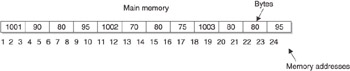Figure 3-4: Elements of a multidimensional array are stored sequentially in memory.

The name of a multidimensional array references the memory address of the first element of the first set of four elements. That is, grades is the equivalent of using memory address 1 in Figure 3-4. You can use the name of a multidimensional array as a pointer to the entire array.

The index of the first element of the first set of array elements points to the memory address where values assigned to array elements are stored.

Referencing the index of the first dimension points to the memory address of the first element of that dimension. For example, referencing grades points to memory address 9 in Figure 3-4. Memory address 9 is the first memory address of contiguous memory where values of the second set of array elements that are associated with grades are stored.

### Declaring a Multidimensional Array

A multidimensional array is declared similar to the way you declare a one-dimensional array except you specify the number of elements in both dimensions. For example, the multidimensional array shown in Figure 3-3 is declared as follows in C or C++:

` int grades; `

The first bracket () tells the compiler that you re declaring 3 pointers, each pointing to an array. This concept might be confusing because the term pointer may make some programmers think of pointer variable or pointer array, which you ll learn about later in this chapter. However, we are not talking about a pointer variable or pointer array. Instead, we are saying that each element of the first dimension of a multidimensional array reference a corresponding second dimension, which is an array.

In this example, all the arrays pointed to by the first index are of the same size. The second index can be of variable size. For example, the previous statement declares a two-dimensional array where there are 3 elements in the first dimension and 4 elements in the second dimension.

The element grades is said to point (just as you use your finger to point) to the second dimension of the array, which is referenced as grades . The second dimension is considered an array. Therefore, programmers say that the first element of a multidimensional array points to another array (that is, the second dimension).

The data type tells the computer that each element of the array will contain an integer data type. The data type is followed by the array name and two values that indicate the size of each dimension used for the array. In this case, there are three sets of four array elements.

You declare a multidimensional array and initialize its elements by using French braces, as shown in Figure 3-5. There are three sets of inner French braces. Each of these sets represents the first dimension of the array. There are four values within each set of inner French braces. These values are assigned to each element of the second dimension of the array.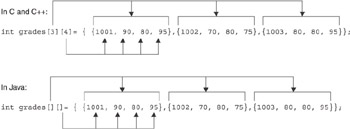Figure 3-5: Braces define sets of values to be assigned to array elements (the top example is C and C++ and the bottom example is Java).

### Assigning Values to a Multidimensional Array

You assign a value to an element of a multidimensional array with an assignment statement similar to the assignment statement that assigns a value to a single-dimensional array, as shown here:

` grades = 1001; `

You must specify the index for both dimensions. In this example, the integer 1001 , which is a student ID, is assigned to the first element of the first set of elements in the grades array.

### Referencing the Contents of a  Multidimensional  Array

The contents of elements of a multidimensional array can be used in a program by referencing the index of both dimensions of the array element. Figure 3-6 shows you how to display the final exam grade for the second student.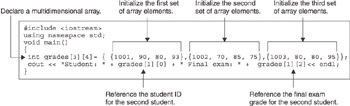Figure 3-6: Display the contents of array elements by referencing the index of both sets of array elements.

In this example, the student ID is displayed by referencing the first element of the second set, and the grade for the final exam is displayed by referencing the third element of the second set.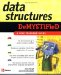Data Structures Demystified (Demystified)
ISBN: 0072253592
EAN: 2147483647
Year: 2006
Pages: 90

Similar book on Amazon